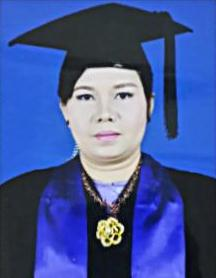Welcome to Department of MathematicsDaw Su Su Hlaing
B.Sc. (Hons;), M.Sc. (Maths)
Department of Mathematics
E.mail.susuhlaing3@mohs.edu.mm

Departmental Objectives

At the end of the first year B. Pharm course in Mathematics, the student should be able to:
• acquire the basic mathematical concepts which will later be useful in its application to medical sciences.
• apply the knowledge of mathematics in solving the problems.
• developed skills in computing methods and techniques associated with information on practical work and research.
• achieve a habit of going through a process of logical thinking by training in mathematical reasoning and thus make correct decisions suitable to circumstances.
• attain basic skills in mathematical modeling and interpretation of results in Medical sciences.

1. U Phoe Doung , M.Sc. (Maths) (2002 – 2005)
2. Daw Aye Kyu , M.Sc. (Maths) ( 2005 – 2009)
3. Daw Phyu Phyu Khin, Ph.D. (Maths) (2009 – 2012)

Total : 0

Total : 0

Total : 0